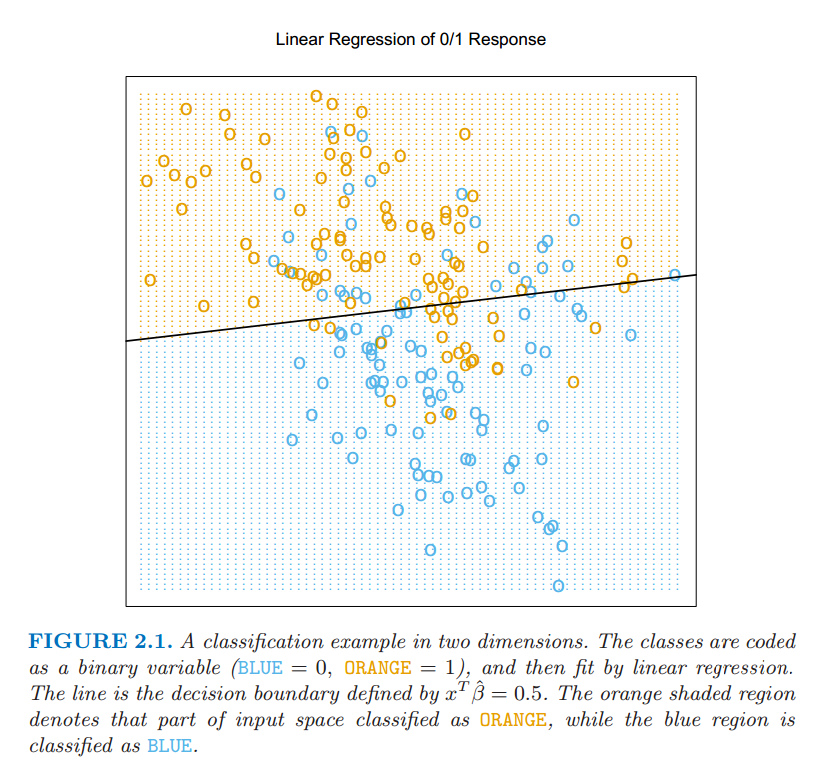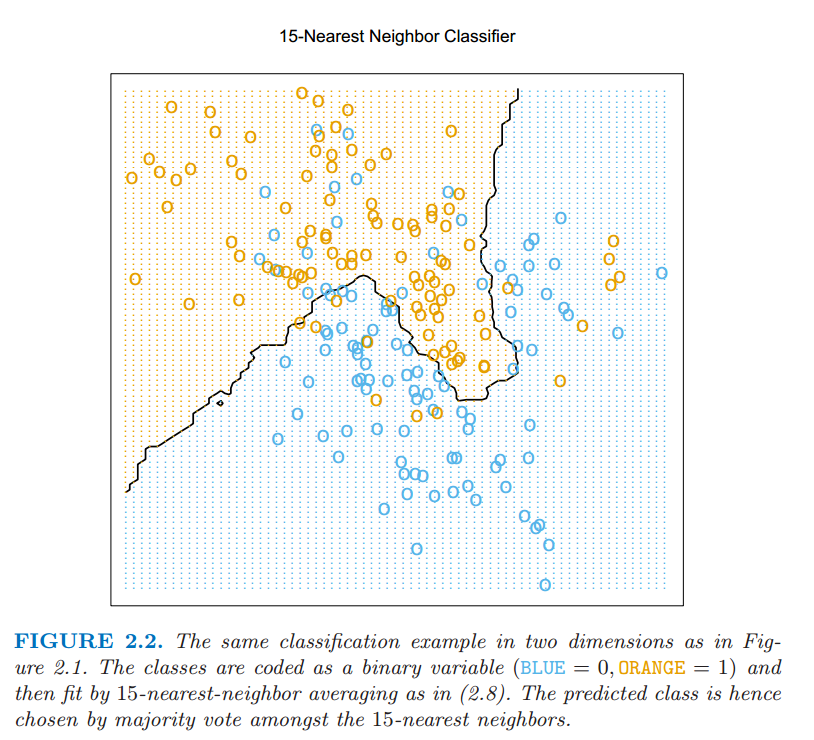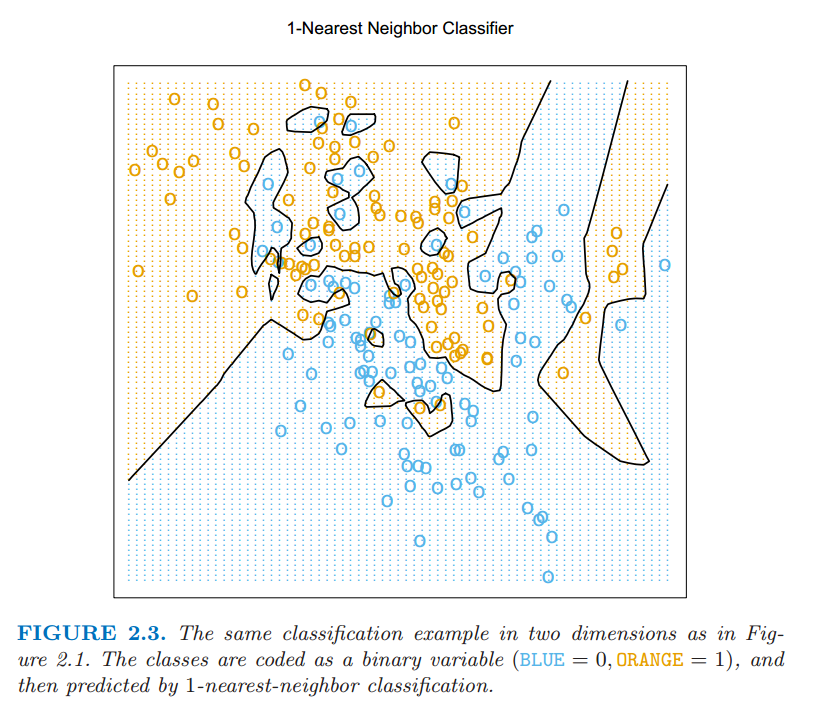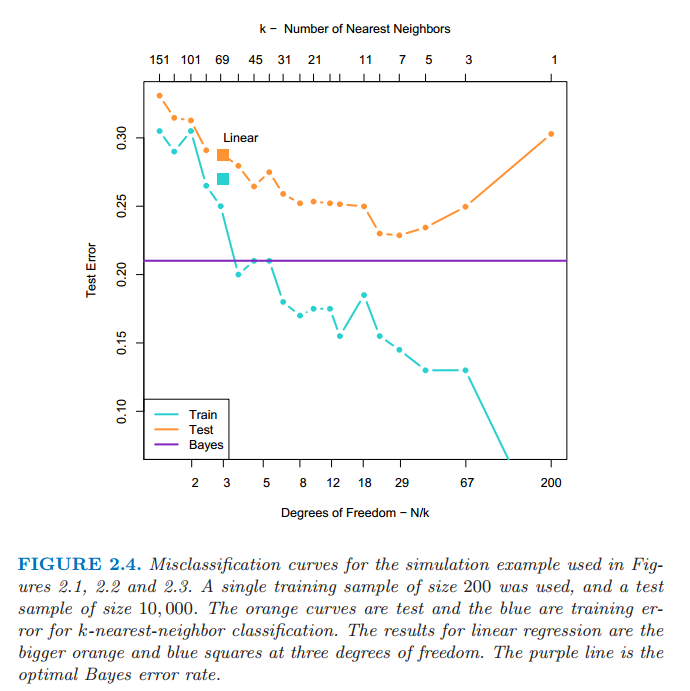# 2.3 两种简单的预测方式：最小二乘和最近邻¶

## 线性模型和最小二乘¶

$\hat{\beta}_0$ 是截距，也是机器学习中的 偏差 (bias)．经常为了方便起见把常数变量 $1$ 放进 $X$，把 $\hat{\beta}_0$ 放进系数变量 $\hat{\beta}$ 中，然后把用向量内积形式写出线性模型

$\RSS(\beta)$ 是系数的二次函数，因此其最小值总是存在，但是可能不唯一．它的解用矩阵来表示是最简单的．我们可以写成## 最邻近方法¶

$N_k(x)$ 是在训练样本中 $k$ 个离 $x$ 最近的点 $x_i$ 组成的邻域．远近意味着度量，目前我们假设是欧氏距离．因此，用文字来叙述就是，我们在输入空间中找到离 $x$ 最近的 $k$ 个观测 $x_i$，然后取这些值的平均．## 从最小二乘到最近邻¶• 核方法使用随着到目标点的距离增大而平滑递减至 $0$ 的权重，而不是像在 $k$-最近邻中用的有效的 $0/1$ 权重系数
• 高维空间中，距离核修改成比其它的变量更多地强调某些变量
• 通过局部权重的最小二乘的局部线性回归，而不是局部用常数值来拟合
• 对原始输入的基函数拓展拟合的线性模型能允许任意复杂的模型
• 投影寻踪和神经网络模型由经过非线性变换的线性模型的总和组成